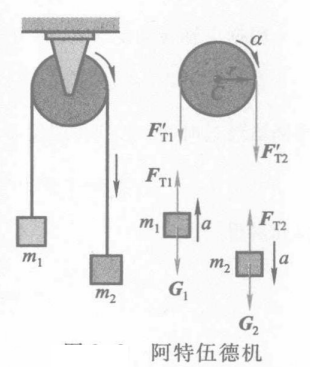\begin{aligned} & F_{\mathrm{T} 1}-G_1=m_1 a \\ & G_2-F_{\mathrm{T} 2}=m_2 a \\ & F_{\mathrm{T} 2}^{\prime} r-F_{\mathrm{T} 1} r=J \alpha \end{aligned}

$$a=r \alpha$$

$$a=\frac{\left(m_2-m_1\right) g}{m_1+m_2+J / r^2}=\frac{\left(m_2-m_1\right) g}{m_1+m_2+m / 2}$$

$$F_{\mathrm{T} 1}=m_1(g+a)=\frac{m_1\left(2 m_2+m / 2\right) g}{m_1+m_2+m / 2}$$

$$\begin{gathered} F_{\mathrm{T} 2}=m_2(g-a)=\frac{m_2\left(2 m_1+m / 2\right) g}{m_1+m_2+m / 2} \\ \alpha=\frac{a}{r}=\frac{\left(m_2-m_1\right) g}{\left(m_1+m_2+m / 2\right) r} \end{gathered}$$

$$F_{\mathrm{T} 1}=F_{\mathrm{T} 2}=\frac{2 m_1 m_2}{m_1+m_2} g, \quad a=\frac{m_2-m_1}{m_2+m_1} g$$